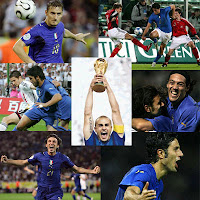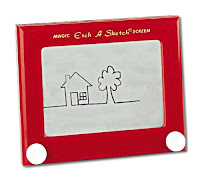## Friday, April 30, 2010

### A circumstantial discoveryAt the conference in Bangalore last week, Jeremy Levy (U. Pittsburgh) gave a fascinating talk about a significant new discovery that allows one to fabricate nanoscale electronic circuits at the interface between two transition metal oxide materials. (as described in this Science paper)

* Jeremy had tickets to the 2006 World Cup Final in Germany. This led to a visit to Augsburg that stimulated the original ideas.

* As a kid, Jeremy had played withEtch A Sketch and understanding how it works inspired the basic idea on how to "write" the circuit onto the interface.

* The original fabrication was not done under vacuum but in air. It turned out that the water vapour present was essential to inducing charge carriers into the interface.

When I started writing this post I thought the discovery involved serendipity, but that involves more when one is looking for something different.

## Thursday, April 29, 2010

### Realisation of a Z2 spin liquid

This follows an earlier post about how spinons and gauge fields emerge in quantum antiferromagnets. It closely follows a review article by Subir Sachdev. Red font highlights important comments.

The magnetic moment for a system with ordering wavevector K [which is incommensurate with the reciprocal lattice] can be written asOne introduces a spinor w, which parameterizes N1,2 by wtoThe Z2 gauge transformationwhere(r,)=1. This Z2gauge invariance plays an important role in stabilising a spin liquid. [Compare this to a commensurate antiferromagnetic where there is a U(1) symmetry associated with the Schwinger bosons].

The idea is to use the Higgs mechanism to reduce the unbroken gauge invariance from U(1) to a discrete gauge group, Z2, and so reduce the strength of the gauge-flux fluctuations. [This is key because U(1) gauge theories are confining in 2+1 dimensions, whereas Z2 can have a deconfined phase]. To break U(1) down to Z2, we need a Higgs scalar,, that carries U(1) charge 2, so thate2iunder the transformation in equation (4). Then a phase withwould break the U(1) symmetry, in the same manner that the superconducting order parameter breaks electromagnetic gauge invariance. However, a gauge transformation with=, while acting non-trivially on the z, would leaveinvariant: thus, there is a residual Z2 gauge invariance that constrains the structure of the theory in the Higgs phase.

What is the physical interpretation of the field?

We see below it desribes deviations of the local magnetic order from the commensurate Neel order.

How does its presence characterize the resulting quantum state, that is, what are the features of this Z2 RVB liquid that distinguish it from other quantum states? The effective action for, constrained only by symmetry and gauge invariance, including its couplings to z, where we expand the theoryto, isThe crucial term is the last one couplinga and z: it indicates thatis a molecular state of a pair of spinons in a spin-singlet state; this pair state has a 'p-wave' structure, as indicated by the spatial gradienta.

The mean-field phase diagram of, as a function of the two 'masses' s and. We have two possible condensates, and hence four possible phases.

(1) s<0,>: this state hasand=0. We may ignore the gappedmodes, and this is just the Néel state

(2) s>0,: this state hasz=0 and=0. Again, we may ignore the gappedmodes, and this is a VBS

(3) s<0,>: this state hasand. Because of the zcondensate, this state breaks spin-rotation invariance, and we determine the spin configuration by finding the lowest-energy zmode in the background of a non-zeroin equation (15), which iswith wbeing a constant spinor. Inserting equation (16) into equation (3), we find thatis space dependent so thatSiobeys equation (6) with N1,2 given by equation (7) and the wavevector K=(,)+2. Thus, this state is a coplanar spin-ordered state. The Z2 gauge transformation in equation (8) is the same as the Z2=transformation.

(4) s>0,: this state hasz=0 and. This is the Z2 spin-liquid (or Higgs) state we are after. Spin-rotation invariance is preserved.